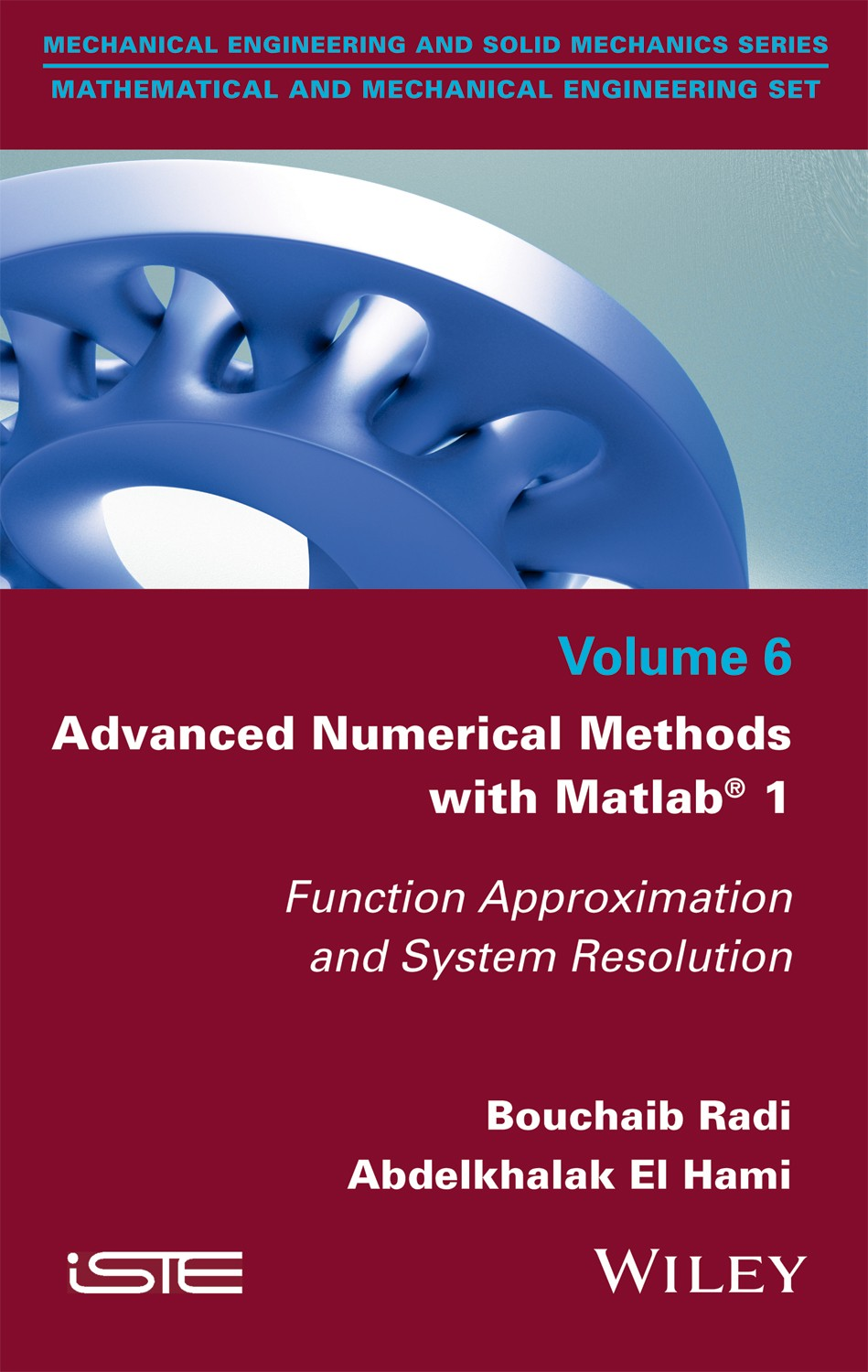pages
238
ISBN
9781786302359

Sorry, this entry is only available in French.

Available at your bookseller and Amazon

Sorry, this entry is only available in French.

Part 1. Introduction
1. Review of Linear Algebra.
2. Numerical Precision.

Part 2. Approximating Functions
3. Polynomial Interpolation.
4. Numerical Differentiation.
5. Numerical Integration.

Part 3. Solving Linear Systems
6. Matrix Norm and Conditioning.
7. Direct Methods.
8. Iterative Methods.
9. Numerical Methods for Computing Eigenvalues and Eigenvectors.
10. Least-squares Approximation.

Part 4. Appendices
Appendix 1. Introduction to Matlab.
Appendix 2. Introduction to Optimization.